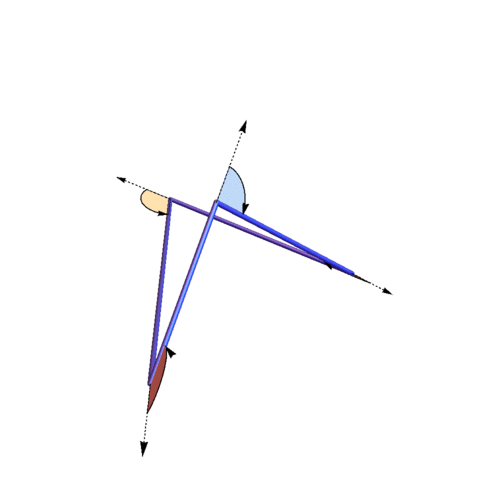# Clayton ShonkwilerIn our paper “The expected total curvature of random polygons”, Jason Cantarella, Alexander Grosberg, Rob Kusner, and I used our previously-defined measure on the space of closed polygons in Euclidean 3-space to compute the exact expectation of total curvature (i.e., the sum of all the turning angles) of such polygons. Specifically, the expected total curvature is exactly $$n \frac{\pi}{2} + \frac{\pi}{4} \frac{2n}{2n-3}$$; previously only the first two terms in the asymptotic expansion of expected total curvature had been known. We also proved a general theorem giving the first two terms in the asymptotic expansion of expected total curvature for any reasonable measure on polygon space.

Shown above is a random 4-gon with the four turning angles marked. The theorem says that the average sum of these four turning angles is exactly $$\frac{12 \pi}{5} \approx$$ 7.53982; for this particular 4-gon the actual total curvature is 7.95492.